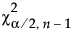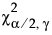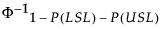d2(n) is the expected value of the range of n independent normally distributed variables with unit standard deviation.
If r is the number of subgroups of size nj and each ith subgroup is defined by the order of the data, sigma is computed as follows:
For a process characteristic with mean μ and standard deviation σ, the population-based capability indices are defined as follows.
LSL is the lower specification limit
USL is the upper specification limit
T is the target value
The 100(1 - α)% confidence interval for Cp is calculated as follows:is the (α/2)th quantile of a chi-square distribution with n - 1 degrees of freedom
n is the number of observations
The 100(1 - α)% confidence interval for Cpk is calculated as follows:
n is the number of observations
The 100(1 - α)% confidence interval for CPM is calculated as follows:is the (α/2)th quantile of a chi-square distribution with γ degrees of freedom
n is the number of observations
T is the target value
s is the long-term sigma estimate
The 100(1 - α)% confidence limits for CPL (denoted by CPLL and CPLU) satisfy the following equations:
tn-1(δ) has a non-central t-distribution with n - 1 degrees of freedom and noncentrality parameter δ
The 100(1 - α)% confidence limits for CPU (denoted by CPUL and CPUU) satisfy the following equations:
tn-1(δ) has a non-central t-distribution with n - 1 degrees of freedom and noncentrality parameter δ
LSL is the lower specification limit
USL is the upper specification limit
T is the target value
Pα is the α*100th percentile of the fitted distribution
For the calculation of Cpm, μ and σ are estimated using the expected value and square root of the variance of the fitted distribution. For more information about the relationship between the parameters in the Parameter Estimates report and the expected value and variance of the fitted distributions, see Continuous Fit Distributions and Discrete Fit Distributions.
LSL is the lower specification limit
USL is the upper specification limit
μ is the sample mean
σ is the sample standard deviationis the (1 - P(LSL) -P(USL))th quantile of a standard normal distribution
P(LSL) = Prob(X < LSL) = 1 - Φ(Z LSL)
P(USL) = Prob(X > USL) = 1 - Φ(Z USL)
Φ is the standard normal cumulative distribution function

Help created on 9/19/2017